# Linear Functions: ax+by+c=0

The general form for a line is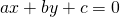whereandare integers. One may rearrange this form to the more familiar slope, y intercept form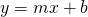.

Example 1: convert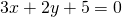toform:Example 2: convertto general form:# Finding the y intercept

The y intercept is the point where the line cuts the y-axis. The x coordinate is zero. To find the y intercept, we substitute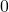for.

Example 3: Find the y intercept of the lineSolution: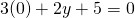Leads us to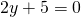Solving we have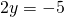and therefore,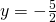# Finding the x intercept

The x intercept is the point that is on the line and on the x axis. The y coordinate is zero. To find the x intercept, we substitutefor.

Example 4: Find the x intercept of the lineSolution:Leads us toSolving we have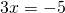and therefore,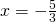# Calculations (Skill #5)

Example: Video

Examples pdf: Standard Form x y int

Calculate the x and y intercepts. Rewrite the equation in the form. Use an integer, a terminating decimal or a fraction for the coefficient of x (that is, don’t round a decimal).